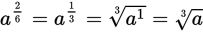# SAT Math Multiple Choice Question 257: Answer and Explanation

### Test Information

Question: 257

2. Which of the following expressions is equivalent to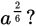• A.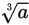• B.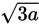• C.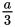• D.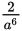Explanation:

A

Difficulty: Easy

Category: Passport to Advanced Math / Exponents

Strategic Advice: A variable with a fraction exponent can be written as a radical expression by writing the numerator of the fraction as the power of the radicand and the denominator as the degree (also called the index) of the root. For example,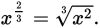Getting to the Answer: Start by reducing the fraction in the exponent: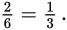The variable a is being raised to the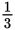power, so rewrite the term as a radical expression with a 3 as the degree of the root and 1 as the power to which a is being raised.